# Math in Focus Grade 7 Chapter 2 Lesson 2.3 Answer Key Multiplying and Dividing Integers

Go through the Math in Focus Grade 7 Workbook Answer Key Chapter 2 Lesson 2.3 Multiplying and Dividing Integers to finish your assignments.

## Math in Focus Grade 7 Course 2 A Chapter 2 Lesson 2.3 Answer Key Multiplying and Dividing Integers

### Math in Focus Grade 7 Chapter 2 Lesson 2.3 Guided Practice Answer Key

Lesson Objective

• Multiply and divide integers.

Hands-On Activity

Explore Multiplication Rules Using Repeated Addition

Work individually.

You can think of multiplying integers as repeated addition.

Step 1
Use a number line to model and complete the multiplication of integers as repeated addition.

a) Evaluate 3 – 2.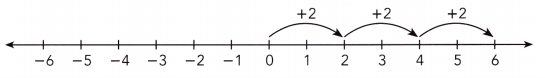3 • 2 means three groups of 2.
3 • 2 = 2 • 3
= 2 + 2 + 2
= 6

Commutative property of multiplication:
Two or more numbers can be multiplied in any order.

b) Evaluate 3 • (-2).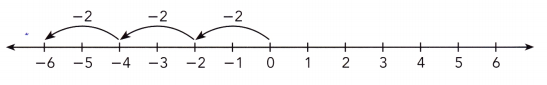3 • (- 2) means three groups of -2.
3 • (-2) = (-2) • 3
= -2 + (-2) + (-2)
=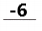The expression 3 • (-2) can also be written as 3(-2).
The expression (-2) • 3 can also be written as -2(3).

Step 2.
Copy and complete the table using repeated addition.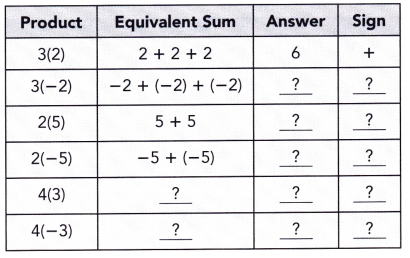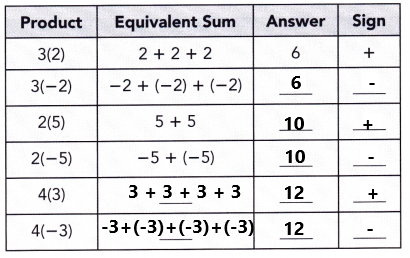Math Journal
Study the pattern in the table from step 2.
a) What do you observe about the sign of the product of two positive integers?
I observed the product of two positive integers with ‘ + ‘ sign.

b) What do you observe about the sign of the product of a positive and a negative integer?
I observed the product of a positive and a negative integer with ‘ – ‘ sign.

Step 3.
Use a number line to model and complete the multiplication as addition of the opposite.

a) Evaluate -3 • 2.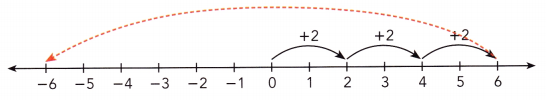You can say that -3 • 2 is the opposite of three groups of 2, 6.
-3 • 2 = -(3)(2)
=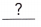I can say that -3 • 2 is the opposite of three groups of 2, 6.
-3 • 2 = -(3)(2)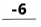b) Evaluate -3 • (-2).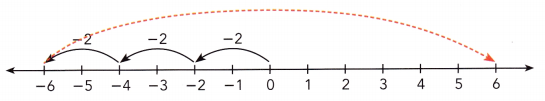You can say that -3 • (-2) is the opposite of three groups of -2, -6.
-3 • (-2) = -(3)(-2)
= -(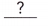)
=I can say that -3 • (-2) is the opposite of three groups of -2, -6.
-3 • (-2) = -(3)(-2)
-(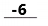)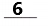Step 4.
Copy and complete the table using addition of the opposite and your results from Step 2.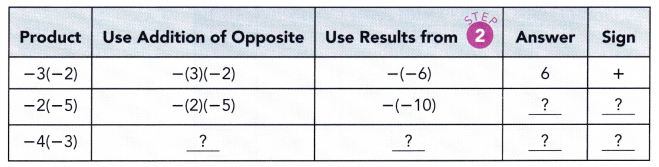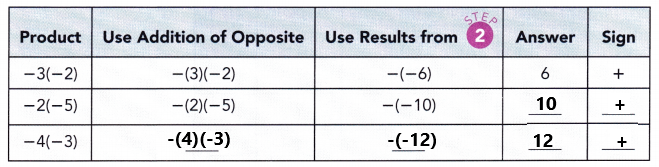Math Journal Based on your observations in Step 1 to Step 4,

a) What do you observe about the sign of the product of two integers with the same sign?
I observed the product of two integers with the same sign results ‘ + ‘ sign.

b) What do you observe about the sign of the product of a positive integer and a negative integer? of integers with different signs?
I observed the product of a positive integer and a negative integer  with ‘ – ‘ sign.

Evaluate each product.

Question 1.
9(-8)
9(-8) = – 72
Explanation:
Perform multiplication operation on above two numbers. Multiply 9 with -8 the product is -72. The product of a positive integer and a negative integer  is represented with ‘ – ‘ sign.

Question 2.
-7 • (-5)
-7 • (-5) = -(7)(-5) = -(-35) = 35
Explanation:
Explanation:
Perform multiplication operation on above two numbers. Multiply -7 with -5 the product is 35. The product of two integers with the same sign results ‘ + ‘ sign.

Question 3.
3(-4)(6)
3(-4)(6) = (-12)(6) = -72
Explanation:
Perform multiplication operation on above three numbers. First multiply 3 with -4 the product is -12. Next multiply -12 with 6 the product is -72. The product of a two positive integers and a negative integer  is represented with ‘ – ‘ sign.

Think Math
Will the product of three negative numbers be positive or negative? What about the product of four negative numbers? Explain your answers.
The product of three negative numbers is negative.
The product of four negative numbers is positive.
Explanation:
Odd numbers of negative integers will have a negative product. Even numbers of negative integers will have a positive product.

Solve.

Question 4.
In a regional golf championship, Steven plays four rounds. The score for a round is recorded as positive (over par) or negative (under par). If Steven scores 6 points under par for all four rounds, what is his total score for his game?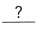• (-6) =His score ispoints.
4 • (-6) = -24
His total score is -24 points.
Explanation:
In a regional golf championship, Steven plays four rounds. It is represented with the number 4. Steven scores 6 points under par for all four rounds. Here under par is represented with ‘-‘ sign. 6 points is represented with -6. Multiply 4 with -6 the product is -24. His total score is -24 points.

Question 5.
The price of a stock falls $2 each day for 9 days. Find the total change in the price of the stock during this time. Answer:$2 • 9 = $18 The price of a stock falls by$18.
Explanation:
The price of a stock falls $2 each day for 9 days. Multiply$2 with 9 the product is $18. The total change in the price of the stock during this time falls by$18.

Evaluate each quotient.

Question 6.
-36 ÷ (-4)
-36 ÷ (-4) = 9
The quotient is 9.
Explanation:
Perform division operation on above two numbers. Divide -36 by -4 the quotient is 9. Even numbers of negative integers will have a positive quotient.

Question 7.
-35 ÷ 5
-35 ÷ 5 = -7
The quotient is -7.
Explanation:
Perform division operation on above two numbers. Divide -35 by 5 the quotient is -7. Odd numbers of negative integers will have a negative quotient.

Question 8.
45 ÷ (-3)
45 ÷ (-3) = -15
The quotient is -15.
Explanation:
Perform division operation on above two numbers. Divide 45 by -3the quotient is -15 Odd numbers of negative integers will have a negative quotient.

Solve.

Question 9.
Find the change in elevation per minute of a hiker who descended 320 feet in 40 minutes.
-320 ÷ 40 = -8 feet/min
Explanation:
A hiker who descended 320 feet in 40 minutes is represented with -320 feet in 40 minutes. The change in elevation per minute of a hiker who descended 320 feet in 40 minutes is -8 feet/min.

### Math in Focus Course 2A Practice 2.3 Answer Key

Evaluate each product.

Question 1.
5 • (-7)
5 • (-7) = -35
Explanation:
Perform multiplication operation on above two numbers. Multiply 5 with -7 the product is -35. The product of a positive integer and a negative integer  is represented with ‘ – ‘ sign.

Question 2.
12 • (-9)
12 • (-9) = -108
Explanation:
Perform multiplication operation on above two numbers. Multiply 12 with -9 the product is -108. The product of a positive integer and a negative integer  is represented with ‘ – ‘ sign.

Question 3.
-6 • 8
-6 • 8 = -48
Explanation:
Perform multiplication operation on above two numbers. Multiply -6 with 8 the product is -48. The product of a negative integer and a positive integer is represented with ‘ – ‘ sign.

Question 4.
-3 • 15
-3 • 15 = -45
Explanation:
Perform multiplication operation on above two numbers. Multiply -3 with 15 the product is -45. The product of a negative integer and a positive integer is represented with ‘ – ‘ sign.

Question 5.
-4 • (-12)
-4 • (-12) = -(4) • (-12) = -(-48) = 48
Explanation:
Perform multiplication operation on above two numbers. Multiply -4 with -12 the product is 48. The product of two integers with the same sign results ‘ + ‘ sign.

Question 6.
-8 • (-20)
-8 • (-20) = -(8) • (-20) = -(-160) = 160
Explanation:
Perform multiplication operation on above two numbers. Multiply -8 with -20 the product is 160. The product of two integers with the same sign results ‘ + ‘ sign.

Question 7.
-14 . 0
-14 • 0 = 0
Explanation:
Perform multiplication operation on above two numbers. Multiply -14 with 0 the product is 0.

Question 8.
0 • (-50)
0 • (-50) = 0
Explanation:
Perform multiplication operation on above two numbers. Multiply 0 with -50 the product is 0.

Question 9.
-3 • 12 • 7
-3 • 12 • 7= (-36)• 7 = -252
Explanation:
Perform multiplication operation on above three numbers. First multiply -3 with 12 the product is -36. Next multiply -36 with 7 the product is -252. The product of a two positive integers and a negative integer is represented with ‘ – ‘ sign.

Question 10.
8 • (-4) • 2
8 • (-4) • 2= (-32)• 2 = -64
Explanation:
Perform multiplication operation on above three numbers. First multiply 8 with -4 the product is -32. Next multiply -32 with 2 the product is -64. The product of a two positive integers and a negative integer is represented with ‘ – ‘ sign.

Question 11.
20 • 5 • (-5)
20 • 5 • (-5) = 100 • (-5)= -500
Explanation:
Perform multiplication operation on above three numbers. First multiply 20 with 5 the product is 100. Next multiply 100 with -5 the product is -500. The product of a two positive integers and a negative integer is represented with ‘ – ‘ sign.

Question 12.
-4 • 10 • (-6)
-4 • 10 • (-6) = -40 • (-6) = 240
Explanation:
Perform multiplication operation on above three numbers. First multiply -4 with 10 the product is -40. Next multiply -40 with -6 the product is -240. The product of a two negative integers and a positive integer is represented with ‘ + ‘ sign.

Question 13.
-7 • (-2) • 10
-7 • (-2) • 10 = 14 • 10 = 140
Explanation:
Perform multiplication operation on above three numbers. First multiply -7 with -2 the product is 14. Next multiply 14 with 10 the product is 140. The product of a two negative integers and a positive integer is represented with ‘ + ‘ sign.

Question 14.
9 • (-6) • (-4)
9 • (-6) • (-4) = (-54) • (-4) = 216
Explanation:
Perform multiplication operation on above three numbers. First multiply 9 with -6 the product is -54. Next multiply -54 with -4 the product is 216. The product of a two negative integers and a positive integer is represented with ‘ + ‘ sign.

Question 15.
-2 • (-8) • (-7)
-2 • (-8) • (-7) = 16 • (-7) = -112
Explanation:
Perform multiplication operation on above three numbers. First multiply -2 with -8 the product is -16. Next multiply 16 with -7 the product is -112. The product of a three negative integers is represented with ‘ – ‘ sign.

Question 16.
-5 • (-12) • (-3)
-5 • (-12) • (-3) = 60 • (-3) = -180
Explanation:
Perform multiplication operation on above three numbers. First multiply -5 with -12 the product is 60. Next multiply 60 with -3 the product is -180. The product of a three negative integers is represented with ‘ – ‘ sign.

Question 17.
14 • 0 • (-15)
14 • 0 • (-15) = 0 • (-15) = 0
Explanation:
Perform multiplication operation on above three numbers. First multiply 14 with 0 the product is 0. Next multiply 0 with -15 the product is 0.

Question 18.
-30 • (-2) • 0
-30 • (-2) • 0 = 60 • 0 = 0
Explanation:
Perform multiplication operation on above three numbers. First multiply -30 with -2 the product is 60. Next multiply 60 with 0 the product is 0.

Question 19.
-6 • (-7) • 2 • 5
-6 • (-7) • 2 • 5
= 42 • 2 • 5
= 84 • 5
= 420
Explanation:
Perform multiplication operation on above three numbers.
First multiply -6 with -7 the product is 42.
Next multiply 42 with 2 the product is 84.
Multiply the obtained product 84 with 5 the product is 420.
The product of a two negative integers and two positive integer is represented with ‘ + ‘ sign.

Question 20.
-8 • (-2) • (-4) • 12
-8 • (-2) • (-4) • 12
= 16 • (-4) • 12
= -64 • 12
= -768
Explanation:
Perform multiplication operation on above three numbers.
First multiply -8 with -2 the product is 16.
Next multiply 16 with 4 the product is -64.
Multiply the above product -64 with 12 the product is -768.
The product of a three negative integers and a positive integer is represented with ‘ – ‘ sign.

Question 21.
-9 • (-5) • (-4) • (-3)
-9 • (-5) • (-4) • (-3)
= 45 • (-4) • (-3)
= -180 • (-3)
= 540
Explanation:
Perform multiplication operation on above three numbers.
First multiply -9 with -5 the product is 45.
Next multiply 45 with -4 the product is -180.
Multiply the above product -180 with -3 the product is 540.
Even numbers of negative integers will have a positive product.

Evaluate each quotient.

Question 22.
125 ÷ (-25)
125 ÷ (-25) = -5
The quotient is -5.
Explanation:
Perform division operation on above two numbers. Divide 125 by -25 the quotient is -5. Odd numbers of negative integers will have negative quotient.

Question 23.
300 ÷ (-15)
300 ÷ (-15) = -20
The quotient is -20.
Explanation:
Perform division operation on above two numbers. Divide 300 by -15 the quotient is -20. Odd numbers of negative integers will have negative quotient.

Question 24.
-100 ÷ 25
-100 ÷ 25 = -4
Explanation:
Perform division operation on above two numbers. Divide -100 by 25 the quotient is -4. Odd numbers of negative integers will have negative quotient.

Question 25.
-32 ÷ 4
-32 ÷ 4 = -8
Explanation:
Perform division operation on above two numbers. Divide -32 by 4 the quotient is -8. Odd numbers of negative integers will have negative quotient.

Question 26.
-480 ÷ (-12)
-480 ÷ (-12) = 40
Explanation:
Perform division operation on above two numbers. Divide -480 by -12 the quotient is 40. Even numbers of negative integers will have positive quotient.

Question 27.
-144 ÷ (-24)
-144 ÷ (-24) = 6
Explanation:
Perform division operation on above two numbers. Divide -144 by -24 the quotient is 6. Even numbers of negative integers will have positive quotient.

Question 28.
0 ÷ (-8)
0 ÷ (-8) = 0
Explanation:
Perform division operation on above two numbers. Divide 0 by -8 the quotient is 0.

Question 29.
0 ÷ (-111)
0 ÷ (-111) = 0
Explanation:
Perform division operation on above two numbers. Divide 0 by -111 the quotient is 0.

Question 30.
While returning to the glider port, Laura descended at minute for 3 minutes. Calculate her change in altitude.

Question 31.
A scuba diver took 6 minutes to rise to the surface at a minute. How far was he below sea level?

Question 32.
An elevator descends 1,500 feet in 60 seconds. Find the change in height per second.
1,500 ÷ 60 = 25 feet/sec
Explanation:
An elevator descends 1,500 feet in 60 seconds. Here descends represented with negative sign. The change in height per second is -25 feet/sec

Question 33.
A scientist measures the change in height per second of a diving osprey as -198 feet per second. Find the change in position of the osprey after 2 seconds.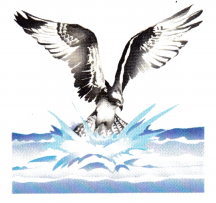The change in height per second of a diving osprey as -198 feet per second.
1 sec = -198 feet
2 sec = ?
-198 • 2 = -396 feet
The change in position of the osprey after 2 seconds is -396 feet.

Question 34.
Math Journal
Margaret wrote -25 ÷ (-100) = $$\frac{-25}{-100}$$ = –$$\left(\frac{1}{4}\right)$$ and
-2 • (-2) = -4. Discuss and correct her mistakes.
-25 ÷ (-100) = 1/4
Perform division operation on above two numbers. Divide -25 by -100 the quotient is 1/4. Even numbers of negative integers will have positive quotient.
-2 • (-2) = 4
Perform multiplication operation on above two numbers. Multiply -2 with -2 the product is 4. The product of two integers with the same sign results ‘ + ‘ sign.

Question 35.
Math Journal Umberto has trouble solving -12 ÷ 3 • 2 ÷ (-4). Write an explanation to help him.